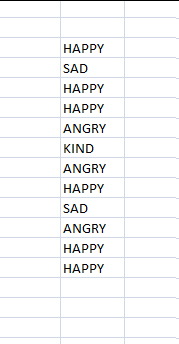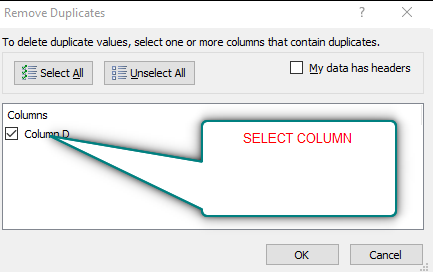PROVIDING ACCURATE, RELIABLE AND STRAIGHT TO THE POINT KNOWLEDGE ABOUT DIFFERENT IT TOOLS EXPLAINED WITH PRACTICAL EXAMPLES

# HOW TO FIND UNIQUE TEXT VALUES IN EXCEL

## INTRODUCTION

Whenever we prepare any report in excel, we have two constituents in any report.

The Text portion and the Numerical portion.

But just storing the text and numbers doesn’t make the super reports. Many times we need to automate the process in the reports to minimize the effort and improve the accuracy.

Many functions are provided by the Excel which work on Text and give us the useful output as well. But few problems are still left on which we need to apply some tricks with the available tools.

THIS WAS AN EXCERPT FROM THE FIRST ARTICLE OF THIS SERIES MANIPULATING TEXT IN EXCEL – PART I

IN THIS ARTICLE, WE’LL LEARN TO COUNT THE UNIQUE TEXT VALUES IN EXCEL.

## HOW TEXT IS HANDLED IN EXCEL?

TEXT is simply the group of characters and strings of characters which convey the information about the different data and numbers in Excel. Every character is connected with a code [ANSI].
Text comprises of the individual entity character which is the smallest bit which would be found in Excel.
We can perform the operations on the strings[Text] or the characters.
Characters are not limited to A to Z or a to z but many symbols are also included in this which we would see in the later part of the article.

TEXT IS AN INACTIVE NUMBER TYPE[FORMAT] IN EXCEL. ANYTHING STORED AS TEXT [NUMBER OR DATE] WON’T RESPOND TO ANY STANDARD FORMULAS OR FUNCTIONS BUT SPECIALLY DESIGNED TEXT FUNCTIONS. [EXCEPTIONS DO OCCUR IN CASE OF NUMBERS]
If we need to make anything inactive, such as Date to be non responding to the calculation, we put it as a text. Similarly if we want to avoid any calculations for a number it needs to be put as a text.

## COUNT UNIQUE TEXT VALUES IN EXCEL

This can be a requirement sometime when we need to count the unique text values in a given data or column. It can be done with a number of ways. Let us discuss a few.

1. USING REMOVE DUPLICATES

2. USING UNIQUE FUNCTION

USING REMOVE DUPLICATES:

This is an option already available in Excel directly and very easy to use.

THIS OPTION IS NOT AUTOMATIC AND SHOULD BE USED ONLY IF WE DON’T HAVE MUCH DATA TO BE ANALYZED.

STEPS TO USE REMOVE DUPLICATES TO COUNT THE UNIQUE TEXT VALUES IN EXCEL

[*KINDLY VISIT HERE FOR COMPLETE INFORMATION ABOUT THE PROCEDURE OF REMOVING DUPLICATES IN EXCEL]

Select the complete data or column where we want to find the number of unique values. [ If we need the original column intact, copy the column and paste it somewhere else. We can perform operations on this column].Go to HOME>DATA>REMOVE DUPLICATES.

Select the column from the dialog box. We have only one column so choose the column.After selecting the column, press OK. It’ll leave only unique values in the column.

Now , we can put the formula =COUNTA(FIRST CELL OF COLUMN: LAST CELL OF COLUMN).

It’ll give us the number of unique text values present in the column.

2. USING UNIQUE FUNCTION
In this method we will make use of UNIQUE FUNCTION which will help us to find out the unique values or texts in the given column. After getting all the unique values we can count them easily. Let us start.
We’ll take an example for trying our procedure.
Suppose we have the following data.STEPS TO COUNT UNIQUE TEXT VALUES IN A EXCEL USING UNIQUE FUNCTION.

[THE COMPLETE PICTORIAL REPRESENTATION OF THE USE OF UNIQUE FUNCTION IS HERE].

We have to process the information twice to get the desired results. First of all we need to find the unique values.

• Select the cell where we want to find the unique values .
• Put the following formula.
• =UNIQUE(ARRAY,COLUMN WISE, FALSE)
• For our example the formula will be
• =UNIQUE(G6:G17,FALSE,0) [The first argument is the array containing the data. The second argument tell it that it has to do the comparison in the rows and third tells that it can count the entries which are more than one].
• The result array will contain only the unique values.
• In the next column we can count these by the use of COUNTA FUNCTION as =COUNTA(H6:H17). We can see in the picture that we had only 4 values but we took a wide range. The reason is that we don’t know what is the number of unique values if the data is too large.
• Take a look at the picture below for the reference.

• ## WHAT IS EXCEL, HOW EXCEL WORKS, WRITE FORMULA IN EXCEL, EXAMPLE OF USING FORMULA IN EXCEL, ANIMATED EXAMLPE OF USING FORMULA IN EXCEL

LEARN

• EXCEL
• JAVASCRIPT
• MORE TO COME…

Encourage us by following at …

If you have any queries or suggestions or any problem which you would like us to solve for you, kindly mail at

[email protected]